# Machine learning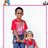Woman scientist Under WOSC Kiran IPR (DST), Qualified Patent Agent, Dean (Innovation) & Professor (CSE) um Rajalakshmi Engineering College Rajalakshmi Nagar, Thandalam Chennai - 602 105
23. Jan 2019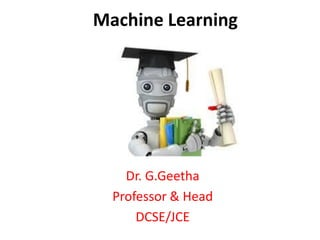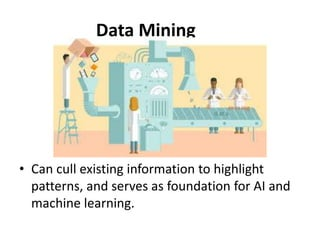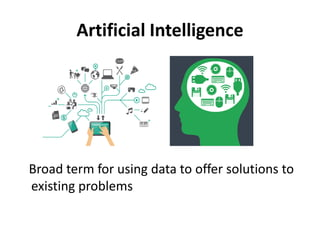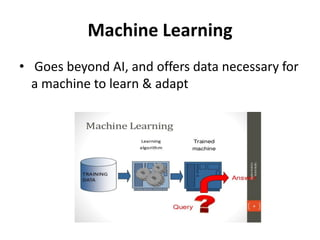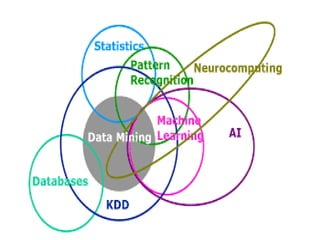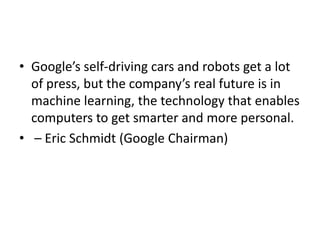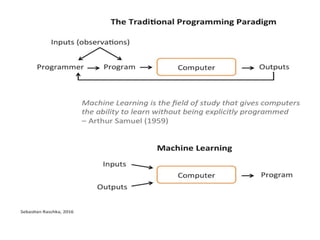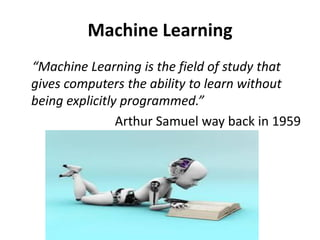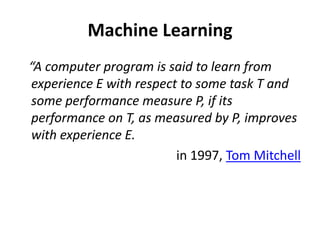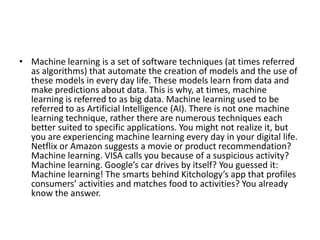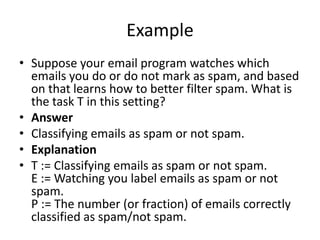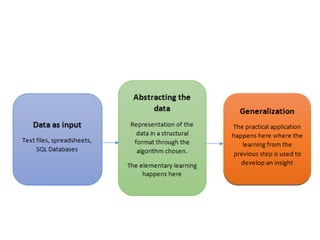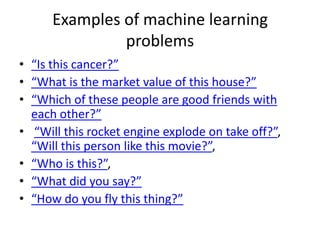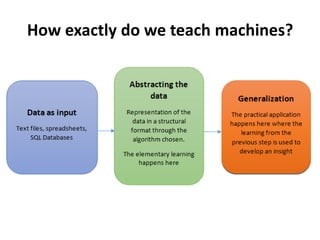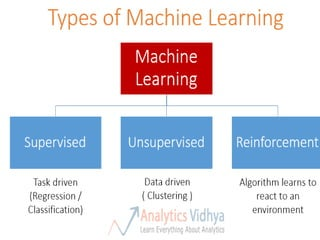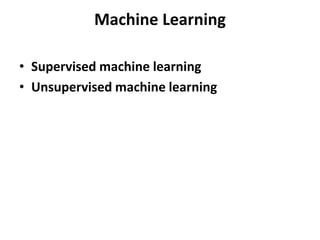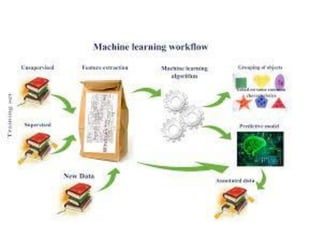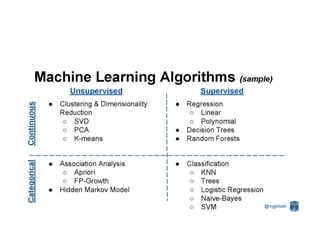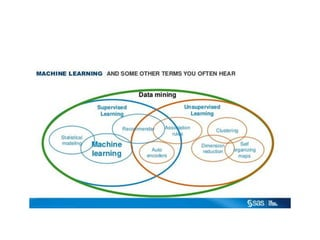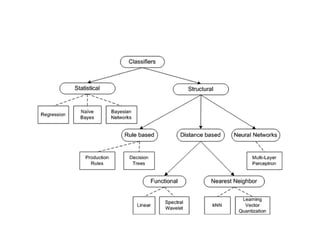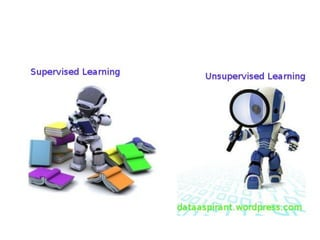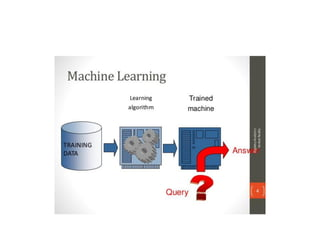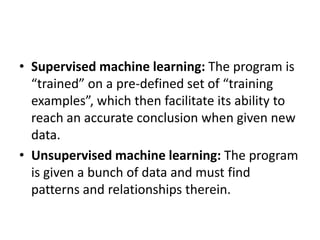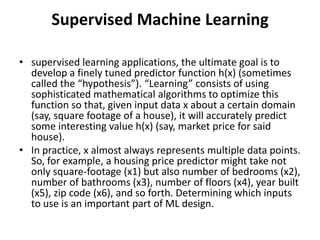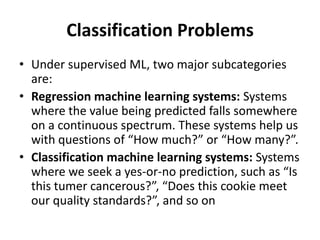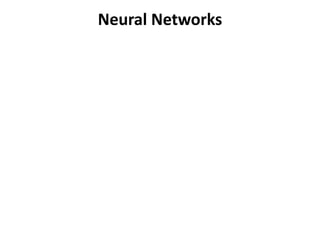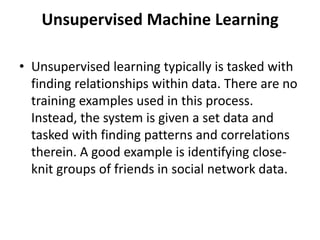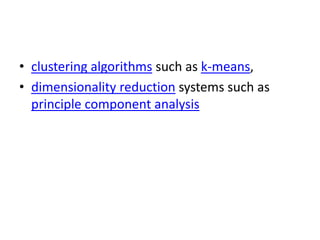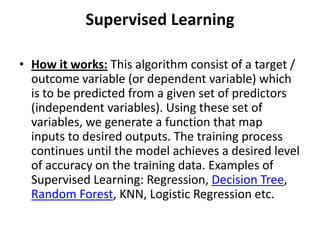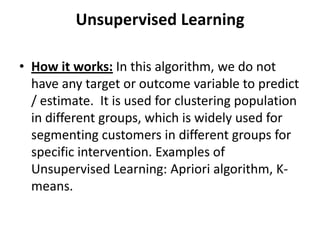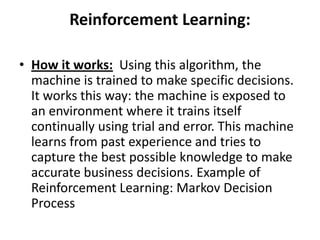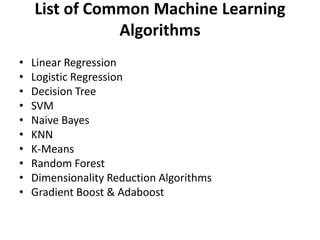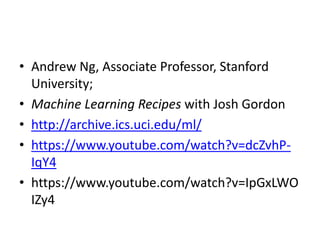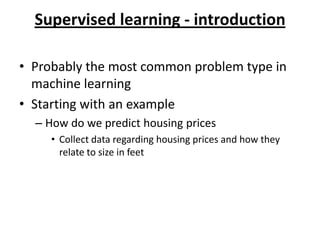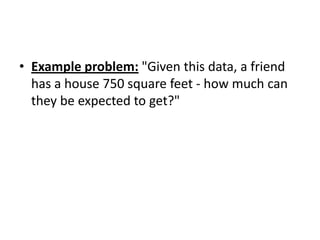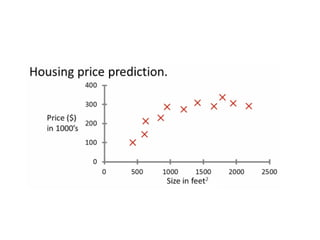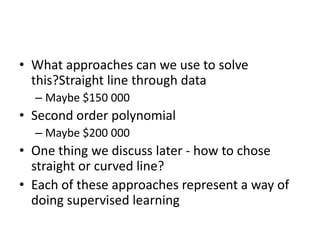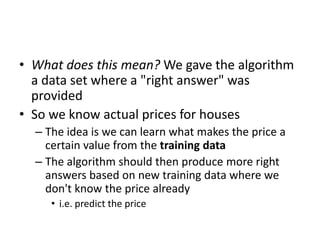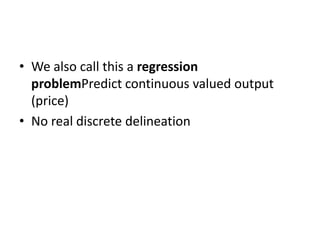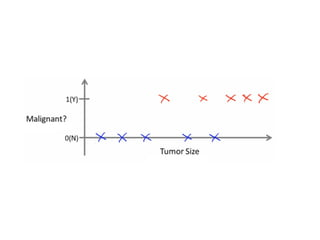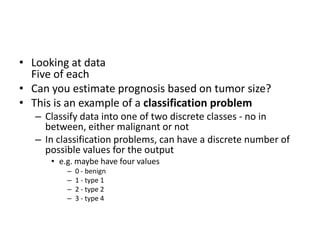1 von 43

### Machine learning

• 2. Data Mining • Can cull existing information to highlight patterns, and serves as foundation for AI and machine learning.
• 3. Artificial Intelligence Broad term for using data to offer solutions to existing problems
• 4. Machine Learning • Goes beyond AI, and offers data necessary for a machine to learn & adapt
• 7. • Google’s self-driving cars and robots get a lot of press, but the company’s real future is in machine learning, the technology that enables computers to get smarter and more personal. • – Eric Schmidt (Google Chairman)
• 9. Machine Learning “Machine Learning is the field of study that gives computers the ability to learn without being explicitly programmed.” Arthur Samuel way back in 1959
• 10. Machine Learning “A computer program is said to learn from experience E with respect to some task T and some performance measure P, if its performance on T, as measured by P, improves with experience E. in 1997, Tom Mitchell
• 11. • Machine learning is a set of software techniques (at times referred as algorithms) that automate the creation of models and the use of these models in every day life. These models learn from data and make predictions about data. This is why, at times, machine learning is referred to as big data. Machine learning used to be referred to as Artificial Intelligence (AI). There is not one machine learning technique, rather there are numerous techniques each better suited to specific applications. You might not realize it, but you are experiencing machine learning every day in your digital life. Netflix or Amazon suggests a movie or product recommendation? Machine learning. VISA calls you because of a suspicious activity? Machine learning. Google’s car drives by itself? You guessed it: Machine learning! The smarts behind Kitchology’s app that profiles consumers’ activities and matches food to activities? You already know the answer.
• 12. Example • Suppose your email program watches which emails you do or do not mark as spam, and based on that learns how to better filter spam. What is the task T in this setting? • Answer • Classifying emails as spam or not spam. • Explanation • T := Classifying emails as spam or not spam. E := Watching you label emails as spam or not spam. P := The number (or fraction) of emails correctly classified as spam/not spam.
• 14. Examples of machine learning problems • “Is this cancer?” • “What is the market value of this house?” • “Which of these people are good friends with each other?” • “Will this rocket engine explode on take off?”, “Will this person like this movie?”, • “Who is this?”, • “What did you say?” • “How do you fly this thing?”
• 15. How exactly do we teach machines?
• 17. Machine Learning • Supervised machine learning • Unsupervised machine learning
• 24. • Supervised machine learning: The program is “trained” on a pre-defined set of “training examples”, which then facilitate its ability to reach an accurate conclusion when given new data. • Unsupervised machine learning: The program is given a bunch of data and must find patterns and relationships therein.
• 25. Supervised Machine Learning • supervised learning applications, the ultimate goal is to develop a finely tuned predictor function h(x) (sometimes called the “hypothesis”). “Learning” consists of using sophisticated mathematical algorithms to optimize this function so that, given input data x about a certain domain (say, square footage of a house), it will accurately predict some interesting value h(x) (say, market price for said house). • In practice, x almost always represents multiple data points. So, for example, a housing price predictor might take not only square-footage (x1) but also number of bedrooms (x2), number of bathrooms (x3), number of floors (x4), year built (x5), zip code (x6), and so forth. Determining which inputs to use is an important part of ML design.
• 26. Classification Problems • Under supervised ML, two major subcategories are: • Regression machine learning systems: Systems where the value being predicted falls somewhere on a continuous spectrum. These systems help us with questions of “How much?” or “How many?”. • Classification machine learning systems: Systems where we seek a yes-or-no prediction, such as “Is this tumer cancerous?”, “Does this cookie meet our quality standards?”, and so on
• 28. Unsupervised Machine Learning • Unsupervised learning typically is tasked with finding relationships within data. There are no training examples used in this process. Instead, the system is given a set data and tasked with finding patterns and correlations therein. A good example is identifying close- knit groups of friends in social network data.
• 29. • clustering algorithms such as k-means, • dimensionality reduction systems such as principle component analysis
• 30. Supervised Learning • How it works: This algorithm consist of a target / outcome variable (or dependent variable) which is to be predicted from a given set of predictors (independent variables). Using these set of variables, we generate a function that map inputs to desired outputs. The training process continues until the model achieves a desired level of accuracy on the training data. Examples of Supervised Learning: Regression, Decision Tree, Random Forest, KNN, Logistic Regression etc.
• 31. Unsupervised Learning • How it works: In this algorithm, we do not have any target or outcome variable to predict / estimate. It is used for clustering population in different groups, which is widely used for segmenting customers in different groups for specific intervention. Examples of Unsupervised Learning: Apriori algorithm, K- means.
• 32. Reinforcement Learning: • How it works: Using this algorithm, the machine is trained to make specific decisions. It works this way: the machine is exposed to an environment where it trains itself continually using trial and error. This machine learns from past experience and tries to capture the best possible knowledge to make accurate business decisions. Example of Reinforcement Learning: Markov Decision Process
• 33. List of Common Machine Learning Algorithms • Linear Regression • Logistic Regression • Decision Tree • SVM • Naive Bayes • KNN • K-Means • Random Forest • Dimensionality Reduction Algorithms • Gradient Boost & Adaboost
• 34. • Andrew Ng, Associate Professor, Stanford University; • Machine Learning Recipes with Josh Gordon • http://archive.ics.uci.edu/ml/ • https://www.youtube.com/watch?v=dcZvhP- IqY4 • https://www.youtube.com/watch?v=IpGxLWO IZy4
• 35. Supervised learning - introduction • Probably the most common problem type in machine learning • Starting with an example – How do we predict housing prices • Collect data regarding housing prices and how they relate to size in feet
• 36. • Example problem: "Given this data, a friend has a house 750 square feet - how much can they be expected to get?"
• 38. • What approaches can we use to solve this?Straight line through data – Maybe \$150 000 • Second order polynomial – Maybe \$200 000 • One thing we discuss later - how to chose straight or curved line? • Each of these approaches represent a way of doing supervised learning
• 39. • What does this mean? We gave the algorithm a data set where a "right answer" was provided • So we know actual prices for houses – The idea is we can learn what makes the price a certain value from the training data – The algorithm should then produce more right answers based on new training data where we don't know the price already • i.e. predict the price
• 40. • We also call this a regression problemPredict continuous valued output (price) • No real discrete delineation
• 41. • Another example – Can we definer breast cancer as malignant or benign based on tumour size
• 43. • Looking at data Five of each • Can you estimate prognosis based on tumor size? • This is an example of a classification problem – Classify data into one of two discrete classes - no in between, either malignant or not – In classification problems, can have a discrete number of possible values for the output • e.g. maybe have four values – 0 - benign – 1 - type 1 – 2 - type 2 – 3 - type 4